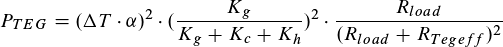# Improving the thermal design of the tracker

31. August 2020 Von eikob

A key point for a good energy income is the thermal design of the harvesting part. We are not only talking about the thermoelectric generator (TEG) itself, but also about its connection to the outer temperature difference. Here, we will briefly showcase the impact of the thermal resistivities on the TEGs power output.

In an ideal world it is easy to calculate the output power of a TEG, especiallywhen you have the datasheet which normally gives you the corresponding seebeck-coefficient α and internal resistance rTEG of your TEG. We are also neglecting any temperature dependencies on these variables as our harvester will only be used in areas relatively small temperature changes and gradients.

Lets shortly think about the basics: You get the output voltage and maximum output power of your TEG as a function of your applied total temperature difference ΔT:

If you are putting a perfectly matched load on your TEG you could theoretically achieve that output:

But reality is a little bit more complex than that. We need a heat flux through the TEG and its thermal connections to the outer temperature difference. All these parts have a thermal resistivity and eat up parts of our temperature difference, we will get directly at our TEG. In fact, a circuit with the TEG embedded between a thermal resistance on both sides can be modelled as an equivalent electrical circuit.

If we than calculate the real temperature difference at the TEG with taking into account our resistances of the TEG Kg, and the hot/cold sides Kh, Kc as an equivalent circuit, we are able to come up to the following formula after some simplification:Output power of a TEG that is embedded between two thermal resistances (Kc and Kh). Attached to it a resistive load Rload. The internal electrical resistance of the TEG RTegeff is normally dependent on the temperature difference directly at the TEG.

This formula gives us the output power – we can see here that not only an electrical impedance matching, but also a thermal one is necessary to achieve maximum output.

But what thermal resistances can we expect when harvesting at an animal? How can we decrease these resistances to get out more power? In one of our papers, we take a closer look at this and try to find rough values for that: https://www.mdpi.com/1996-1073/13/11/2769